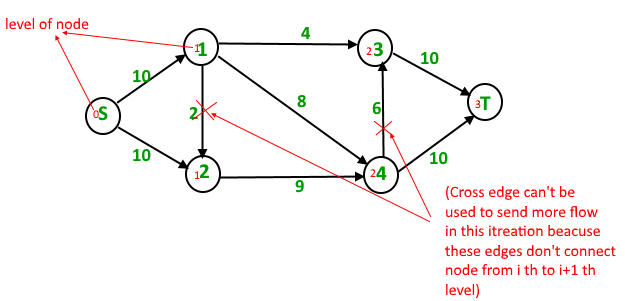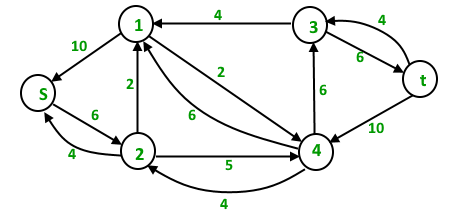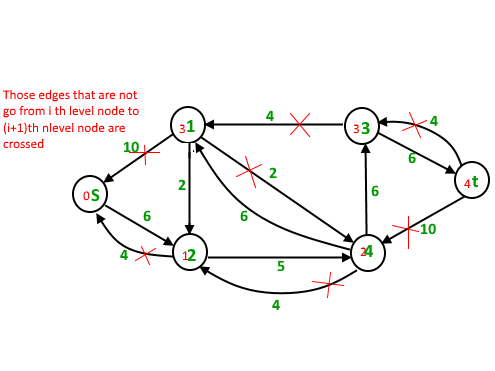Related Articles

# Dinic’s algorithm for Maximum Flow

• Difficulty Level : Expert
• Last Updated : 02 Feb, 2021

Problem Statement :
Given a graph which represents a flow network where every edge has a capacity. Also given two vertices source ‘s’ and sink ‘t’ in the graph, find the maximum possible flow from s to t with following constraints :

1. Flow on an edge doesn’t exceed the given capacity of the edge.
2. Incoming flow is equal to outgoing flow for every vertex except s and t.

For example, in following input graph,the maximum s-t flow is 19 which is shown below.Background :

1. Max Flow Problem Introduction : We introduced Maximum Flow problem, discussed Greedy Algorithm and introduced residual graph.
2. Ford-Fulkerson Algorithm and Edmond Karp Implementation : We discussed Ford-Fulkerson algorithm and its implementation. We also discussed residual graph in detail.

Time complexity of Edmond Karp Implementation is O(VE2). In this post, a new Dinic’s algorithm is discussed which is a faster algorithm and takes O(EV2).

Like Edmond Karp’s algorithm, Dinic’s algorithm uses following concepts :

1. A flow is maximum if there is no s to t path in residual graph.
2. BFS is used in a loop. There is a difference though in the way we use BFS in both algorithms.

In Edmond’s Karp algorithm, we use BFS to find an augmenting path and send flow across this path. In Dinic’s algorithm, we use BFS to check if more flow is possible and to construct level graph. In level graph, we assign levels to all nodes, level of a node is shortest distance (in terms of number of edges) of the node from source. Once level graph is constructed, we send multiple flows using this level graph. This is the reason it works better than Edmond Karp. In Edmond Karp, we send only flow that is send across the path found by BFS.

Outline of Dinic’s algorithm :

```1) Initialize residual graph G as given graph.
1) Do BFS of G to construct a level graph (or
assign levels to vertices) and also check if
more flow is possible.
a) If more flow is not possible, then return.
b) Send multiple flows in G using level graph
until blocking flow is reached. Here using
level graph means, in every flow,
levels of path nodes should be 0, 1, 2...
(in order) from s to t.```

A flow is Blocking Flow if no more flow can be sent using level graph, i.e., no more s-t path exists such that path vertices have current levels 0, 1, 2… in order. Blocking Flow can be seen same as maximum flow path in Greedy algorithm discussed discussed here.

Illustration :
Initial Residual Graph (Same as given Graph)Total Flow = 0

First Iteration : We assign levels to all nodes using BFS. We also check if more flow is possible (or there is a s-t path in residual graph).Now we find blocking flow using levels (means every flow path should have levels as 0, 1, 2, 3). We send three flows together. This is where it is optimized compared to Edmond Karp where we send one flow at a time.
4 units of flow on path s – 1 – 3 – t.
6 units of flow on path s – 1 – 4 – t.
4 units of flow on path s – 2 – 4 – t.
Total flow = Total flow + 4 + 6 + 4 = 14
After one iteration, residual graph changes to following.Second Iteration : We assign new levels to all nodes using BFS of above modified residual graph. We also check if more flow is possible (or there is a s-t path in residual graph).Now we find blocking flow using levels (means every flow path should have levels as 0, 1, 2, 3, 4). We can send only one flow this time.
5 units of flow on path s – 2 – 4 – 3 – t
Total flow = Total flow + 5 = 19
The new residual graph isThird Iteration : We run BFS and create a level graph. We also check if more flow is possible and proceed only if possible. This time there is no s-t path in residual graph, so we terminate the algorithm.

Implementation :
Below is c++ implementation of Dinic’s algorithm:

## CPP

 `// C++ implementation of Dinic's Algorithm``#include``using` `namespace` `std;` `// A structure to represent a edge between``// two vertex``struct` `Edge``{``    ``int` `v ;  ``// Vertex v (or "to" vertex)``             ``// of a directed edge u-v. "From"``             ``// vertex u can be obtained using``             ``// index in adjacent array.` `    ``int` `flow ; ``// flow of data in edge` `    ``int` `C;    ``// capacity` `    ``int` `rev ; ``// To store index of reverse``              ``// edge in adjacency list so that``              ``// we can quickly find it.``};` `// Residual Graph``class` `Graph``{``    ``int` `V; ``// number of vertex``    ``int` `*level ; ``// stores level of a node``    ``vector< Edge > *adj;``public` `:``    ``Graph(``int` `V)``    ``{``        ``adj = ``new` `vector[V];``        ``this``->V = V;``        ``level = ``new` `int``[V];``    ``}` `    ``// add edge to the graph``    ``void` `addEdge(``int` `u, ``int` `v, ``int` `C)``    ``{``        ``// Forward edge : 0 flow and C capacity``        ``Edge a{v, 0, C, adj[v].size()};` `        ``// Back edge : 0 flow and 0 capacity``        ``Edge b{u, 0, 0, adj[u].size()};` `        ``adj[u].push_back(a);``        ``adj[v].push_back(b); ``// reverse edge``    ``}` `    ``bool` `BFS(``int` `s, ``int` `t);``    ``int` `sendFlow(``int` `s, ``int` `flow, ``int` `t, ``int` `ptr[]);``    ``int` `DinicMaxflow(``int` `s, ``int` `t);``};` `// Finds if more flow can be sent from s to t.``// Also assigns levels to nodes.``bool` `Graph::BFS(``int` `s, ``int` `t)``{``    ``for` `(``int` `i = 0 ; i < V ; i++)``        ``level[i] = -1;` `    ``level[s] = 0;  ``// Level of source vertex` `    ``// Create a queue, enqueue source vertex``    ``// and mark source vertex as visited here``    ``// level[] array works as visited array also.``    ``list< ``int` `> q;``    ``q.push_back(s);` `    ``vector::iterator i ;``    ``while` `(!q.empty())``    ``{``        ``int` `u = q.front();``        ``q.pop_front();``        ``for` `(i = adj[u].begin(); i != adj[u].end(); i++)``        ``{``            ``Edge &e = *i;``            ``if` `(level[e.v] < 0  && e.flow < e.C)``            ``{``                ``// Level of current vertex is,``                ``// level of parent + 1``                ``level[e.v] = level[u] + 1;` `                ``q.push_back(e.v);``            ``}``        ``}``    ``}` `    ``// IF we can not reach to the sink we``    ``// return false else true``    ``return` `level[t] < 0 ? ``false` `: ``true` `;``}` `// A DFS based function to send flow after BFS has``// figured out that there is a possible flow and``// constructed levels. This function called multiple``// times for a single call of BFS.``// flow : Current flow send by parent function call``// start[] : To keep track of next edge to be explored.``//           start[i] stores  count of edges explored``//           from i.``//  u : Current vertex``//  t : Sink``int` `Graph::sendFlow(``int` `u, ``int` `flow, ``int` `t, ``int` `start[])``{``    ``// Sink reached``    ``if` `(u == t)``        ``return` `flow;` `    ``// Traverse all adjacent edges one -by - one.``    ``for` `(  ; start[u] < adj[u].size(); start[u]++)``    ``{``        ``// Pick next edge from adjacency list of u``        ``Edge &e = adj[u][start[u]];``                                    ` `        ``if` `(level[e.v] == level[u]+1 && e.flow < e.C)``        ``{``            ``// find minimum flow from u to t``            ``int` `curr_flow = min(flow, e.C - e.flow);` `            ``int` `temp_flow = sendFlow(e.v, curr_flow, t, start);` `            ``// flow is greater than zero``            ``if` `(temp_flow > 0)``            ``{``                ``// add flow  to current edge``                ``e.flow += temp_flow;` `                ``// subtract flow from reverse edge``                ``// of current edge``                ``adj[e.v][e.rev].flow -= temp_flow;``                ``return` `temp_flow;``            ``}``        ``}``    ``}` `    ``return` `0;``}` `// Returns maximum flow in graph``int` `Graph::DinicMaxflow(``int` `s, ``int` `t)``{``    ``// Corner case``    ``if` `(s == t)``        ``return` `-1;` `    ``int` `total = 0;  ``// Initialize result` `    ``// Augment the flow while there is path``    ``// from source to sink``    ``while` `(BFS(s, t) == ``true``)``    ``{``        ``// store how many edges are visited``        ``// from V { 0 to V }``        ``int` `*start = ``new` `int``[V+1] {0};` `        ``// while flow is not zero in graph from S to D``        ``while` `(``int` `flow = sendFlow(s, INT_MAX, t, start))` `            ``// Add path flow to overall flow``            ``total += flow;``    ``}` `    ``// return maximum flow``    ``return` `total;``}` `// Driver Code``int` `main()``{``    ``Graph g(6);``    ``g.addEdge(0, 1, 16 );``    ``g.addEdge(0, 2, 13 );``    ``g.addEdge(1, 2, 10 );``    ``g.addEdge(1, 3, 12 );``    ``g.addEdge(2, 1, 4 );``    ``g.addEdge(2, 4, 14);``    ``g.addEdge(3, 2, 9 );``    ``g.addEdge(3, 5, 20 );``    ``g.addEdge(4, 3, 7 );``    ``g.addEdge(4, 5, 4);` `    ``// next exmp``    ``/*g.addEdge(0, 1, 3 );``      ``g.addEdge(0, 2, 7 ) ;``      ``g.addEdge(1, 3, 9);``      ``g.addEdge(1, 4, 9 );``      ``g.addEdge(2, 1, 9 );``      ``g.addEdge(2, 4, 9);``      ``g.addEdge(2, 5, 4);``      ``g.addEdge(3, 5, 3);``      ``g.addEdge(4, 5, 7 );``      ``g.addEdge(0, 4, 10);` `     ``// next exp``     ``g.addEdge(0, 1, 10);``     ``g.addEdge(0, 2, 10);``     ``g.addEdge(1, 3, 4 );``     ``g.addEdge(1, 4, 8 );``     ``g.addEdge(1, 2, 2 );``     ``g.addEdge(2, 4, 9 );``     ``g.addEdge(3, 5, 10 );``     ``g.addEdge(4, 3, 6 );``     ``g.addEdge(4, 5, 10 ); */` `    ``cout << ``"Maximum flow "` `<< g.DinicMaxflow(0, 5);``    ``return` `0;``}`

Output:

`Maximum flow 23`

Time Complexity : O(EV2). Doing a BFS to construct level graph takes O(E) time. Sending multiple more flows until a blocking flow is reached takes O(VE) time. The outer loop runs at-most O(V) time. In each iteration, we construct new level graph and find blocking flow. It can be proved that the number of levels increase at least by one in every iteration (Refer the below reference video for the proof). So the outer loop runs at most O(V) times. Therefore overall time complexity is O(EV2).

References :
https://en.wikipedia.org/wiki/Dinic’s_algorithm